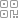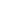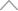6 0 8 2 1 8 8

【Excel基础篇】Excel公式的运算顺序9+2^4

=9-(-2)^4

=int((A4+4)*6)

=(3+2)x[2+(10-4)÷3]+3

2

=(3+2)*(2+(10-4)/3)+3^2

新知精选

优选课程 新知学院

•扫码下载商业新知APP~

•回到顶部